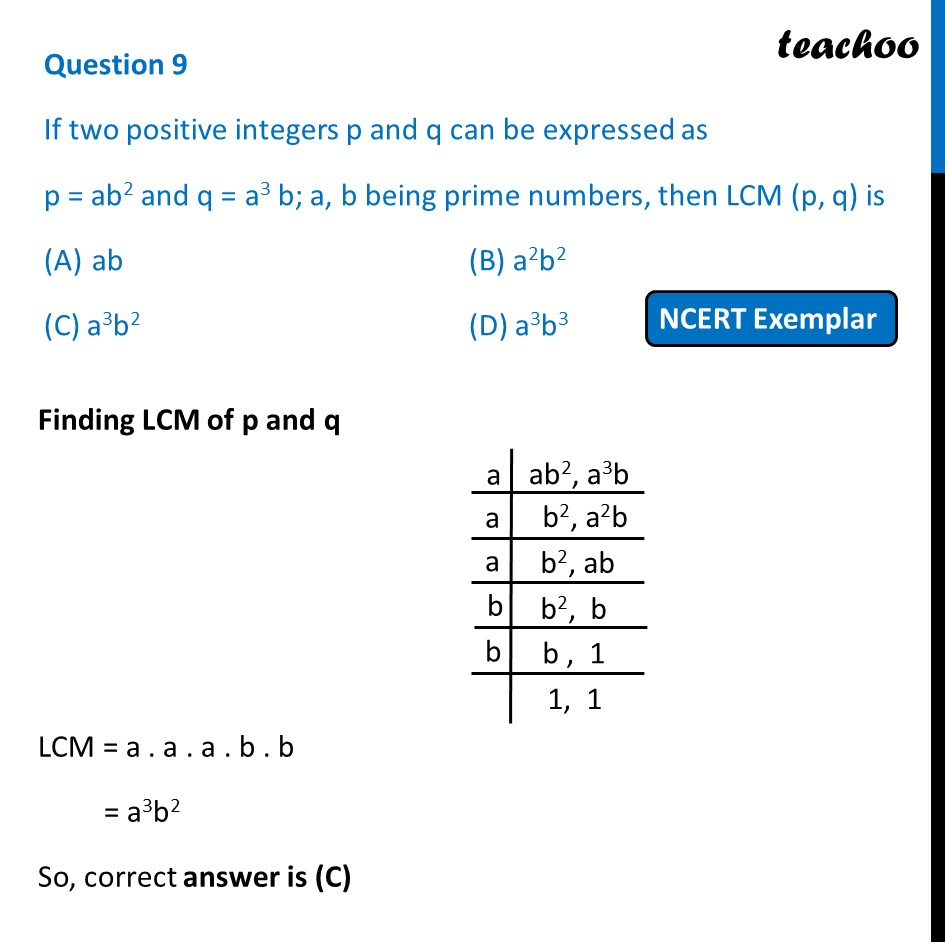## (C) a 3 b 2          (D) a 3 b 31. Chapter 1 Class 10 Real Numbers (Term 1)
2. Serial order wise
3. MCQs from NCERT Exemplar

Transcript

Question 9 If two positive integers p and q can be expressed as p = ab2 and q = a3 b; a, b being prime numbers, then LCM (p, q) is ab (B) a2b2 (C) a3b2 (D) a3b3 Finding LCM of p and q LCM = a . a . a . b . b = a3b2 So, correct answer is (C)

MCQs from NCERT Exemplar